### IMO Shortlist 1997 problem 12

Kvaliteta:
Avg: 0,0
Težina:
Avg: 0,0
Let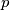$p$ be a prime number and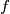$f$ an integer polynomial of degree$d$ such that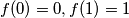$f(0) = 0,f(1) = 1$ and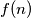$f(n)$ is congruent to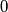$0$ or$1$ modulo$p$ for every integer$n$. Prove that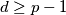$d\geq p - 1$.
Izvor: Međunarodna matematička olimpijada, shortlist 1997